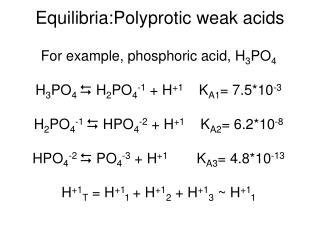DownloadDownload PresentationEquilibria:Polyprotic weak acids

# Equilibria:Polyprotic weak acids

Download Presentation## Equilibria:Polyprotic weak acids

- - - - - - - - - - - - - - - - - - - - - - - - - - - E N D - - - - - - - - - - - - - - - - - - - - - - - - - - -
##### Presentation Transcript

1. Equilibria:Polyprotic weak acids For example, phosphoric acid, H3PO4 H3PO4  H2PO4-1 + H+1 KA1= 7.5*10-3 H2PO4-1 HPO4-2 + H+1 KA2= 6.2*10-8 HPO4-2  PO4-3 + H+1 KA3= 4.8*10-13 H+1T = H+11 + H+12 + H+13 ~ H+11

2. Equilibria: Salts Illustrated using a simple =1/-1 salt There are 4 reactions to consider. • CatAn  Cat+1 + An-1 • Cat+1 + H2O  CatOH + H+1 KA = KW/KB • An-1 + H2O  Han + OH-1 KB = KW/KA • H2O  H+1 + OH-1 KW

3. Equilibria: Salts We may classify salts into 4 groups, depending on the source of the cation and anion. SBSA (NaCl), WBSA (NH4Cl), SBWA (NaF), WB WA (NH4F). The pH of the final solution will be influenced by one or more of the previous reactions.

4. Equilibria: Salts WAWB salts are especially interesting. The pH is influenced by both the cation and anion hydrolysis reactions. • Cat+1 + H2O  CatOH + H+1 KA = KW/KB • An-1 + H2O  Han + OH-1 KB = KW/KA The pH of the final solution will depend on the size of KA & KB. KA >KB, acidic, etc.

5. Equilibria: Titrations If one titrates an acetic acid solution with a sodium hydroxide solution. There are 4 stages in the titration. • Only acetic acid, pH = -log(CAKA)/2 • Buffer, pH = pKA + log [A-] [HA] • WASB salt, pOH = -log(CAKB)/2 note, must find new “acid” concentration • Strong base pH = 14 - pOH

6. Equilibria: Solubility & Solubility Products If a salt is “insoluble” or sparingly soluble, one may write an equilibrium expression for the reaction with water. MXAY(S)  XM+Y(aq) + YA-X(aq) KSP = [M+Y]X[A-X]Y e.g. CaF2(S) Ca+2(aq) + 2F-1(aq) KSP = [Ca+2]1[F-1]2 KSP = 4.2*10-11

7. Equilibria: Solubility & Solubility Products We prepare a solution which is 1.0*10-4M in copper(I) and 2.0*10-3M in lead(II). To this solution we slowly add sodium iodide. Which will precipitate first, CuI or PbI2? The KSP’s are respectively, 5.3*10-12 and 1.4*10-8.